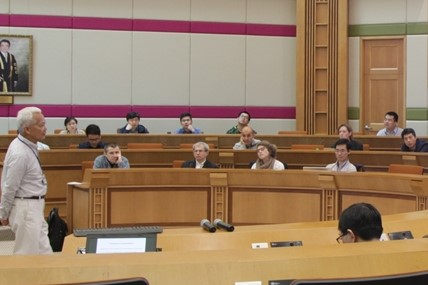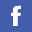## Colloquium/Seminar

 Year Month 2022 Jan     Feb     Mar     Apr     May     Jun 2021 Feb     Mar     Apr     May     Jun     Jul     Aug     Sep     Oct     Nov     Dec 2020 Jan     May     Jun     Jul     Aug     Sep     Oct     Nov     Dec 2019 Jan     Feb     Mar     Apr     May     Jun     Jul     Aug     Oct     Nov 2018 Jan     Feb     Mar     Apr     May     Jun     Jul     Aug     Oct     Nov     Dec

### Event(s) on March 2018

• Tuesday, 6th March, 2018

• Wednesday, 7th March, 2018

 Title: TVD-based Finite Volume Methods for Sound-Advection-Buoyancy Systems Speaker: Prof. Dr. Joerg Wensch, Technical University Dresden, Germany Time/Place: 11:30  -  12:30 FSC1217, Fong Shu Chuen Library, HSH Campus, Hong Kong Baptist University Abstract: The simulation of atmospheric dynamics is an important issue in Numerical Weather Prediction. It relies on the numerical solution of the Euler equations. In this talk we provide an overview on the history of numerical weather prediction as well as an introduction to splitting techniques for the Euler equations. The Euler equations exhibit phenomena on different temporal scales. In the lower troposphere sound waves propagate approximately ten times faster than the advective waves. We present multirate infinitesimal step (MIS) schemes based on a finite volumes spatial discretization with different treatment of slow and fast processes in the time discretization. An approach to overcome the CFL restriction caused by sound waves are split-explicit methods. Through multirate techniques the terms relevant for sound waves are integrated by small time steps with a cheap time integration procedure, whereas the slow processes are solved by an underlying Runge-Kutta method using a larger macro step size. The analysis of these methods is based on the interpretation as an exponential or Lie group integrator. By assuming an exact solution of the fast waves with frozen coefficients for the slow waves order conditions for our multirate infinitesimal step methods are derived. Stability is discussed with respect to the linear acoustics equation. We construct methods based on TVD-RK schemes by different search and optimization procedures. For the established RK3 time stepping scheme the stability bound with respect to the sound CFL number is approximately 3. For our methods this bound extends up to 12. Numerical simulation results for established benchmark problems are presented. The theoretically predicted properties are confirmed by the experiments

• Tuesday, 20th March, 2018

 Title: Least Squares Piecewise Monotonic Data Approximation: Algorithms and Applications Speaker: Prof. Ioannis Constantine Demetriou, Department of Mathematics and Informatics, Department of Economic, National and Kapodistrian University of Athens, Greece Time/Place: 11:30  -  12:30 FSC1217, Fong Shu Chuen Library, HSH Campus, Hong Kong Baptist University Abstract: Algorithms and applications are presented for the following data approximation problem. Let phi_i: i=1,2,...,n} be measurements of smooth function values {f(x_i): i=1,2,...,n}, where the measurements are so rough that the number of sign changes in the sequence {phi_{i+1}-phi_{i}: i=1,2,...,n-1}, say it is sigma, is much greater than the number in the sequence {f(x_{i+1})-f(x_{i}): i=1,2,...,n-1}. The problem of calculating the least squares change to the data subject to the condition that the first differences of the estimated values have at most k-1 sign changes is considered, where k is a prescribed integer. The estimates form a n-vector with k monotonic sections in its components, alternately increasing and decreasing. The main difficulty in this optimization calculation is that the optimal positions of the joins of the monotonic sections have to be found automatically, but the number of all possible combinations of positions can be of magnitude n^{k-1}, so that it is not practicable to test each one separately. It is shown that the case when k=1 is straightforward, and that the case when k>1 reduces to partitioning the data into at most k disjoint sets of adjacent data and solving a k=1 problem for each set. It is shown also that the partition into suitable sets can be done by a dynamic programming method, which can be made quite efficient by taking advantage of some properties that are implied by the optimization calculation. Two methodologically different algorithms have been developed. The first one requires O(knsigma) computer operations. The other algorithm reduces the complexity to O(nsigma+ksigmalog_2sigma) operations, when k >= 3, by taking advantage of some ordering relations between the indices of the joins during the data partition process. The complexity is only O(n) when k=1 or 2. In relation to these algorithms, Fortran software packages have been written by the author and some of their numerical results will be given. The packages can manage routinely very large amounts of data. For example, they require few seconds to calculate a best fit with 10 or 100 monotonic sections to 30000 very noisy data on a common pc. The piecewise monotonicity approximation method may have many applications, in various contexts in several disciplines. For example, it is highly suitable in estimating the turning points (peaks) of a function from some noisy measurements of its values.Peak finding is a subject of continuous interest in spectroscopy, chromatography and signal processing. Other examples arise from medical imaging, from signal processing and from data analysis for instance. A selection of application results will be presented.

• Tuesday, 27th March, 2018

 Title: A Tour in Combinatorics and Graph Theory Speaker: Prof. Weifan Wang, Zhejiang Normal University, China Time/Place: 16:00  -  17:30 (Preceded by Reception at 3:45pm) RRS905, Sir Run Run Shaw Building, HSH Campus, Hong Kong Baptist University Abstract: Combinatorics and graph theory is the science of studying discrete objects. With the rapid development of computer science, the study of combinatorics and graph theory becomes more and more important. Besides important applications in computer science; communication; coding and cryptography; physics; chemistry; and biology, it can be directly applied to enterprise management; transportation; war command; and financial analysis. In this talk, we shall focus on classical problems in combinatorics and graph theory including the four color problem; the seven bridges problem; Hamiltonian cycle problem; the shortest path problem; Ramsay number; pigeonhole principle; and Fibonacci sequence. We shall introduce their backgrounds, history, development and applications.

All years### Conferences and Workshops

We organize conferences and workshops every year. Hope we can see you in future.### Lecture Series

Details### Employment